# What is the Fermi Dirac distribution function

## f. What are the distribution functions in many particle systems?

A very important question in statistical mechanics is how the entire energy of the system is distributed to the individual particles. Or in other words: what are the energies of the individual particles?

The distribution functions were developed to answer this question. In the section on bosons and fermions it was already mentioned that the nature of the particles is decisive for the distribution function. Consequently there are different distribution functions for bosons, fermions and classical particles.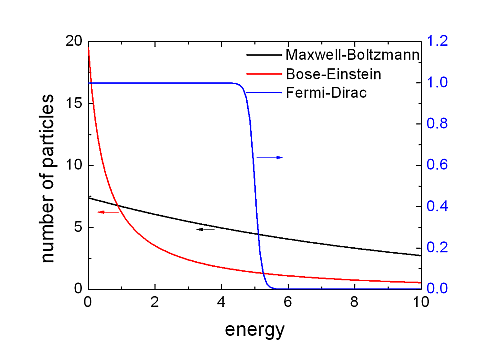A good example of a classic many-particle system is a gas made up of atoms or molecules under standard conditions. Its energy distribution can be described using the Boltzmann statistics.

The almost free electron gas in an electrical conductor under standard conditions can be viewed as an example of a fermionic system. Its energy distribution is described by the Fermi-Dirac distribution.

The photon gas, a black body, mentioned in the last section, can be used as a last example of a bosonic system. Its energy distribution is described by the Bose-Einstein distribution.

The figure above shows the 3 distribution functions. With the help of a little math, you can better illustrate their differences.

The Boltzmann distribution is given by the formula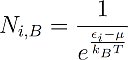described. With this formula the number Ni of the particles with energy εi in a gas with temperature T and chemical potential μ in thermal equilibrium. It is noticeable that Ni can also be smaller than one.

It has already been mentioned several times that the theories presented here are statistical in nature. So there is a number less than one for Ni the probability an, a particle with energy εi to be found. Ni= 0.4 means that there is a 40% chance of finding a particle with this energy. If it is one, you will definitely find a particle with this energy and at Ni greater than one accordingly several. Another special feature is that the energies are plotted on a standardized scale. So energy means zero, medium energy. Values ​​not equal to zero describe a deviation from the mean value. With the Bose-Einstein and the Boltzmann distribution, one finds more particles in the mean energy than in all other energies. While in the Fermi-Dirac distribution only a single particle can be found there. This is a consequence of the fact that two fermions can never have the same quantum state.

In the same way the distribution function for fermions can be: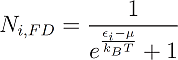And the one for bosons: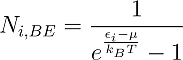write down. The indices B, FD and BE stand for Boltzmann, Fermi-Dirac and Bose-Einstein.

One can easily see that the only difference between the distribution functions is the +1 and -1 in the denominator. But that changes everything. For example, in the drawing above you can see the Ni, FD never gets bigger than one. This is because the denominator can never be less than one because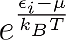is always greater than one. So in a fermionic many-particle system two particles can never have the same energy, unless they differ in a further quantum number (e.g. the spin). However, this should not be discussed further at this point, as it is not important for further discussion.

Based on the above drawing, it seems that the difference between Bose-Einstein and Boltzmann statistics is only qualitative and not quantitative. That this is not true can be seen in the next section, which finally revolves around the question:

What is Bose Einstein Condensation?